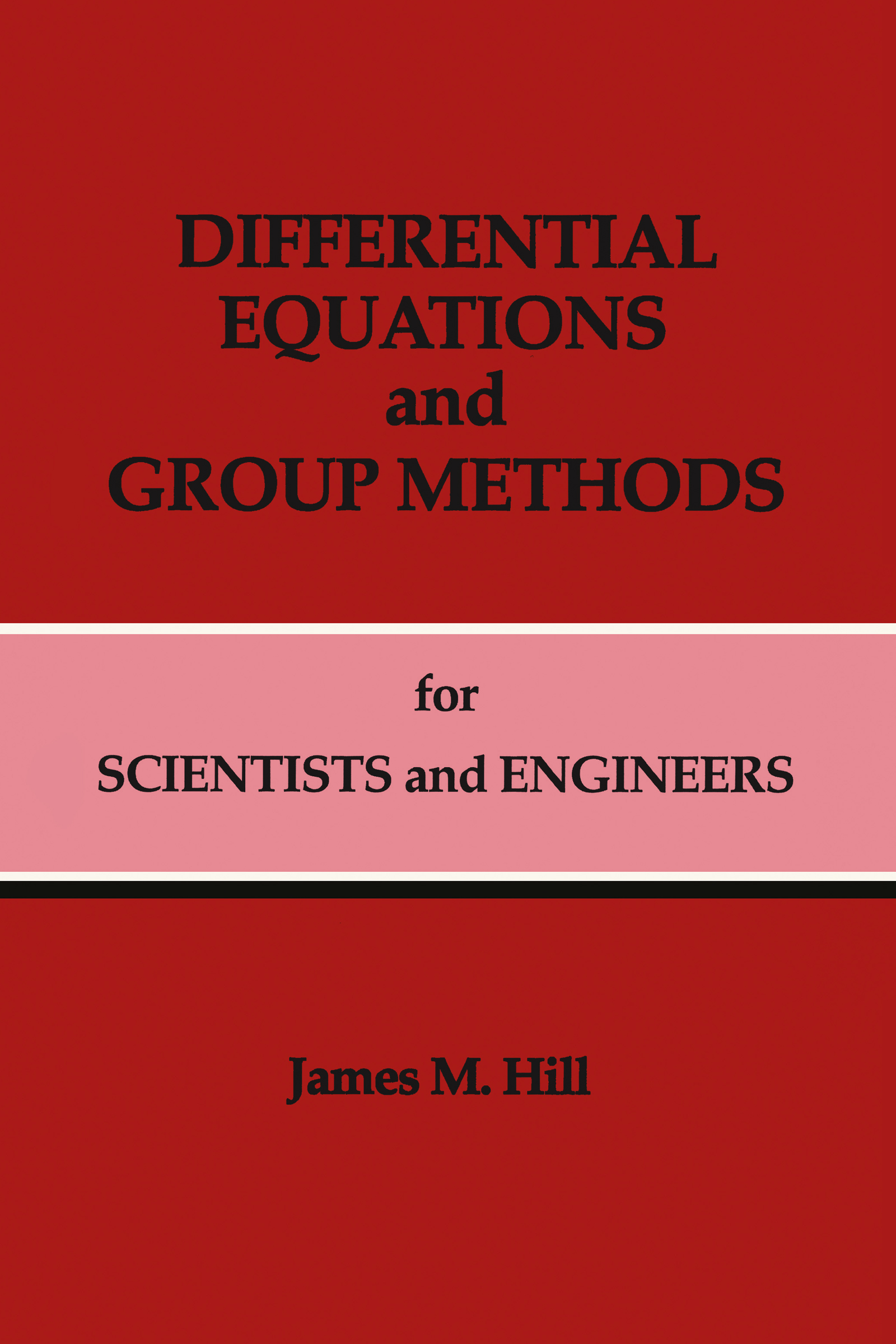# Differential Equations and Group Methods for Scientists and Engineers

## 1st Edition

CRC Press

224 pages

##### Purchasing Options:\$ = USD
Hardback: 9780849344428
pub: 1992-03-17
SAVE ~\$48.00
Currently out of stock
\$240.00
\$192.00
x

FREE Standard Shipping!

### Description

Differential Equations and Group Methods for Scientists and Engineers presents a basic introduction to the technically complex area of invariant one-parameter Lie group methods and their use in solving differential equations. The book features discussions on ordinary differential equations (first, second, and higher order) in addition to partial differential equations (linear and nonlinear). Each chapter contains worked examples with several problems at the end; answers to these problems and hints on how to solve them are found at the back of the book. Students and professionals in mathematics, science, and engineering will find this book indispensable for developing a fundamental understanding of how to use invariant one-parameter group methods to solve differential equations.

### Table of Contents

PREFACE. INTRODUCTION. ONE-PARAMETER GROUPS AND LIE SERIES. One- INVARIANCE OF STANDARD LINEAR ORDINARY DIFFERENTIAL EQUATIONS. First Order Equation g'=p(x)g=q(x). FIRST ORDER ORDINARY DIFFERENTIAL EQUATIONS. SECOND AND HIGHER ORDER ORDINARY DIFFERENTIAL EQUATIONS. LINEAR PARTIAL DIFFERENTIAL EQUATIONS. NON-LINEAR PARTIAL DIFFERENTIAL EQUATIONS. BIBLIOGRAPHY. ANSWERS AND HINTS. SUMMARY OF RESEARCH AREAS.

### Subject Categories

##### BISAC Subject Codes/Headings:
MAT000000
MATHEMATICS / General
MAT003000
MATHEMATICS / Applied
MAT007000
MATHEMATICS / Differential Equations